# Colleges with the lowest SAT scores in Arizona

Top 7 colleges in Arizona with the lowest SAT scores
Looking for the colleges with the lowest SAT scores in Arizona? Well you're in luck! We've compiled a national college database and have created a list of the top 7 universities with the lowest SAT scores in Arizona below. If you are not a good test taker or worried about your test scores, this list is for you. These are the schools whose applicants had the lowest average SAT scores in Arizona, which means that you can get into these colleges with a lower SAT score. We also include each college's ACT scores and acceptance rate so that you can see where you would have the easiest time getting in. Read on to find out more.

## Grand Canyon University SAT scores

The average SAT score for Grand Canyon University is 1000.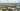The average SAT score of 1000 breaks down into:

• SAT math: 500

The average ACT score for Grand Canyon University is 19 and their acceptance rate is 67%.

## University of Advancing Technology SAT scores

The average SAT score for University of Advancing Technology is 1035.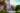The average SAT score of 1035 breaks down into:

• SAT math: 510

The average ACT score for University of Advancing Technology is 21 and their acceptance rate is 83%.

## Northern Arizona University SAT scores

The average SAT score for Northern Arizona University is 1130.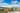The average SAT score of 1130 breaks down into:

• SAT math: 560

The average ACT score for Northern Arizona University is 23 and their acceptance rate is 82.6%.

## Prescott College SAT scores

The average SAT score for Prescott College is 1150.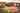The average SAT score of 1150 breaks down into:

• SAT math: 550

The average ACT score for Prescott College is 24 and their acceptance rate is 79.6%.

## University of Arizona SAT scores

The average SAT score for University of Arizona is 1196.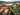The average SAT score of 1196 breaks down into:

• SAT math: 610

The average ACT score for University of Arizona is 25 and their acceptance rate is 84.4%.

## Arizona State University SAT scores

The average SAT score for Arizona State University is 1250.The average SAT score of 1250 breaks down into:

• SAT math: 630

The average ACT score for Arizona State University is 26 and their acceptance rate is 84.8%.

## Arizona Western College SAT scores

The average SAT score for Arizona Western College is 1300.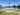The average SAT score of 1300 breaks down into:

• SAT math: 650